# Is my PWM fan controller design good?

#### TheMasterXXXXX

Joined Aug 23, 2022
29
I am new to circuit design, and thought it would be an interesting project to develop an analog fan controller for 2 wire fans, with programmable temperature settings. If you are willing to take the time to read this description, I would very much appreciate any feedback or insights regarding the design.

The temperatures will be selected by two, 6 position slide switches, with the 6 positions labeled 40°C-90°C. One switch will be labeled 'Low Temperature', and the other 'High Temperature' (described in detail below). The device has two modes, 'PWM' and 'Schmidt Trigger':

• Schmidt Trigger - The user selects an 'On' temperature with the 'High Temperature' switch, and an 'Off' temperature with the 'Low Temperature' switch. When the temperature probe exceeds the selected high temperature, the fan will turn on. It will turn off when the temperature falls below the selected low temperature.
• PWM - The fan will turn on, at minimal power, when the selected low temperature is reached. From that point, it will increase in power as temperature increases, and will reach max power when the selected high temperature is reached.
The device accepts an input voltage of 5V-24V, with the voltage regulation designed as follows: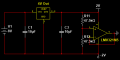The op-amp output is used as a virtual ground, 2V and -2V are used as rails for the rest of the circuit.

Temperature Probe schematic: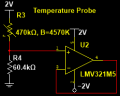The probe is comprised of a thermistor based voltage divider and a voltage follower. A graphical representation of the output voltage as a function of temperature can be seen here. At 40°C, the output voltage is 0.42V. At 90°C, it is 1.33V.

Voltage Reference schematic: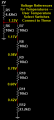The five voltages referenced here represent the voltage output of the temperature probe at temperatures 40°C-90°C. They will later be utilized in comparison operations.

Schmitt Trigger schematic: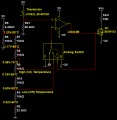Note: The thermistor voltage follower is omitted in this image

Initially, the temperature probe output is compared with the High Temperature voltage reference. If the probe voltage is greater, the op-amp output goes high, turning on the fan and toggling the SPDT analog switch. Now, the temperature probe is compared to the Low Temperature voltage reference. If the probe voltage falls below the reference, the fan will turn off, and the voltage being compared against will again be the High Temperature voltage.

PWM Controller:

I generate a 4Vpp triangular wave with two op-amps, using the method described here: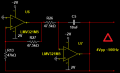I now relate the measured temperature to the high and low temperatures selected by the user: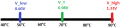In this image, the measured temperature is half way between the selected low and high temperatures, so the fan should run at 50% power (50% duty cycle).

Next, I normalize this value (50% in this example) over the peak to peak range of the triangular wave (+2V — -2V). 50% between +2V — -2V is 0V. I feed this result, together with the triangular wave, into a comparator. I then use the comparator output (which will be high 50% of the time) to drive a MOSFET, protected by this TVS diode.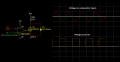I achieve the normalization over the triangular wave range by computing the following formula: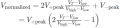Computing this formula involves dividing by a calculated voltage (V_high-V_low). My aim was to avoid using costly components such as voltage multiplier/divider ICs, so I implemented the following solution: Both temperature select switches are DP6T, each acting as depicted below (only with 6 positions instead of 5):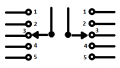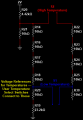On the right side, the difference in position between the high temperature and low temperature switches is represented by the series of resistors between the two switch contacts. In the image above, this amounts to 4 resistors (40kΩ). Using this series of resistors, I can now alter the normalization formula accordingly (CONTINUED IN NEXT POST):

Last edited:

#### TheMasterXXXXX

Joined Aug 23, 2022
29
New normalization formula: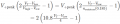I then solve the formula using four op-amps, as follows: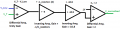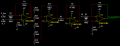These are the components used:
• All resistors are 0.1% tolerance
• Thermistor R0 = 470kΩ 1%, B= 4570K 1%
• Op-amps
• Analog Switch
• Voltage Regulator
• MOSFET

The circuit seems to work in the SPICE simulation. I have not yet constructed it using actual parts.
Once again, much thanks if you have taken the time to review this. If not, documenting the circuit in such a way has in any event been helpful to me.

Last edited: